Is QT paradoxical? Log Out | Topics | Search Moderators | Edit Profile

 The Peoples' Book Forum » On the Failings of Reason (Archived) » Is QT paradoxical? « Previous Next »

Author Message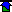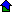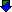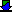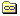Israel
 Posted on Thursday, July 10, 2008 - 10:02 pm: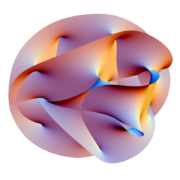(Calabi-Yau 3D manifold)
Algebraic Geometry

The theory which we call Quantum Mechanics is very strange.
Because when we are talking about mechanics it means that we
can imagine and see this process visually. But QM came with
no visual aids, no model to picture in one's mind. Now this
theory is a purely mathematical formalism, difficult to use
and impossible to visualize. It simply gives the right
answers to the most complicated theoretical question. Such
situation satisfy maybe 99% of physicists. But there are few
physicists who donâ€™t agree with this situation. They want to
understand QT without paradoxes. I consider that these
paradoxes are connected with only one reason: " Nobody pays
attention to the geometrical form of particles".
Now the physicists follow "pure" mathematicians. "Since the
mathematical physicists have taken over, theoretical physics
has gone to pot. The bizarre concepts generated out of the
over use and misinterpretation of mathematics would be funny
if it were not for the tragedy of the waste in time,
manpower, money, and the resulting misdirection."- - said
Richard Feynman. There is difference between "pure"
mathematics and the mathematics of theoretical physics. "
Pure" mathematics is infinite and the mathematics of
theoretical physics is limited by natural laws. The "pure"
mathematicians have all rights to create and use abstract
models ( point, line â€¦etc) Physicists must use mathematical
apparatus in connection with real objects, with real

1.In thermodynamics particles are "mathematical points",

2. In QT particles are "mathematical points",

3. In SRT, particles are points. But according SRT
the "mathematical point", cannot be a firm "mathematical
point" . It means it is a "elastic point", which can change
its form. (??!!).

4. When this "mathematical elastic point" flies with speed
c=1 its form become a flat circle, not a " mathematical
point" flying with speed c=1.

5. In QED an electron is an
elastic sphere, which can change its form. (??!!).

6. The power, impulse, linear and angular momentum in
physics is also a " mathematical point".

7. Then one "mathematical point" /particle/ interacts with
another "mathematical point" / power, impulse / the
physicists say: " The micro-world is paradoxical."

8. If physicists think about a particle as a " mathematical
point" the result can be only paradoxical. And I am sure if
somebody takes into consideration the geometrical form of
particle the paradoxes of QT will disappear.
-------------------------------------------------------------..

P.S.
In an Italian railway station:
It was more then two hours 'till the departure of the train.
I went to the cafĂ© and ordered a cup of coffee. Soon two men
and a very beautiful, slim woman took a place opposite me.
They ordered something to drink and one of the man opened a
case of violin and took out a bow. He began to explain
something about the bow, carefully and gently touching it.
Then another man took this bow and also enthusiastically
continued this conversation. For half an hour the bow was
passed from one hands to another followed with enthusiastic
discussion. And the beautiful woman looked at bow and the
both these men without saying a word. For half an hour I
watched this group with admiration and excitement. What a
class! What a cultural level! What a beauty!
And now let's imagine the bow pressed into a "mathematical
point" and the musicians speak seriously about
a "mathematical point" which must produce a sound from a
violin. Everybody will say I describe an idiotic situation.
Well, I agree. But why doesn't anybody say it to physicists
when they observe an elementary particle as a "mathematical
point" , without paying attention to its geometrical form.
When Feynman said "I think I can safely say that nobody
understands quantum mechanics." it was only because nobody
took into consideration the geometrical form of a particle.
==================...

Best wishes.

Israel

www.socratus.com

(posted via email to humancafe.com)Israel
 Posted on Friday, July 11, 2008 - 07:53 am:

Physics and Geometry. / My opinion./
--------------------.
The Classic Physics was started from two points:
thermodynamics and light.
1.
By studying the effect of thermodynamics, physicists came to the
opinion that the physical parameters like volume, temperature and
density (of particles mass) are enough to discover the laws of
thermodynamics, and they didn’t need to know something
concrete about single particle. But then, soon or later, all mass of this
particles will stop it’s moving and the thermal/ radiation death
will come. Is it possible? No, it isn’t , some particles will radiate
and then QT evolved from this idea. Now the situation radically change.
From studying mass of particles in thermodynamics Planck and Einstein
began to study one individual particle (quantum of energy). It seams that
it was logical to think about geometrical form of this particle, but this
did not happen. And still now physicists do not think about concrete
particles, they are concerned about the “mathematical point”.
2.
When physicists studied the behavior of light, they came to the
conclusion that light ( light quanta) can sometime be a particle as a
“ mathematical point” and sometime a wave as a “ mathematical
wave". From behavior of light the SRT was born and here the particle
is also “ mathematical point”. It is hard to understand, why nobody think
about geometrical form of light quanta if it is real particle.
3.
For many years, physicists used Euclidian (static and firm ) geometry
for solving physical problems, and they thought there was only one geometry.
But Lobachevsky and Bolyai had another opinion.
They thought that to use only Euclidian geometry was not enough to
explain all the effects in the Universe. Why, because our Universe is not
static and firm. The physical processes in Universe change all the time
so the Euclidian geometry also has to change. This lead Lobachevsky and
Bolyai to discover Non-Euclidian geometry which is not static but elastic.
4.
Between the XIX and XX century, many physicists such Abraham,
Poincare, Lorentz and Einstein came to the conclusion that the particle
(electron) does not have constant mass, energy and length. This means
that an electron is not a firm particle. The electron is an elastic particle
and therefore his geometrical form can change. All physicists know about
this fact and took this fact in their calculations. But which conclusion can
be done from this fact? They have no answer. Nobody interested about
the borders of this changes.
5.
In 1915 Einstein said the mass and speed (moving mass) can change the
geometry of space. (GRT). It means the physics without geometry is a
limited part of science. It means the physics without geometry is not
complete (whole) part of science.
6.
The situation we see today is similar to the years between 1900 and
1928 when QT was created. Nothing changes.
Mathematicians use Non- Euclidian geometry and they do not know
the power of these changes from Euclidian to Non-Euclidian geometry.
They do not interested how these changes came.
And physicists use forces (energy, impulse, …etc) without know
this. For them the particle is only represented by a “mathematical point”.
After all, they say the situation in QT is crazy, the Nature is paradoxical.
I know why they say this, because one hand “physical” doesn’t know that
the other hand “geometrical” does.
7.
Can we understand our “ paradoxical” world?
The answer is clear. In the Natural world, physics and geometry
are one unit part in the evolutionary process, and this fact must be
reflected in any future theory of the evolution of matter (as a mass
an as a individual particle).
8.
There are two ways to reflect this process.
One way explained by Einstein in GRT as a man who observed
the situation from outside.
Another way can be explained by Lobachevsky / Bolyai geometry
using it in conjunction with the inner (!) impulse of Planck-Einstein (h)
and the inner (!) impulse of Goudsmit – Uhlenbeck (h=h/2pi)..

Questions:
1) Where does the Planck/Einstein factor h come from?
2) Where does the Goudsmit / Uhlenbeck factor h=h/2pi come from?
3) What is the physical difference between h and h=h/2pi in the Nature.
4) Can the process of evolution go without geometrical changes?
Without the physical/ geometrical theory we cannot answer these questions.
-----------------------.
And on my opinion, only when we begin to think about light quanta as
a particle with a geometrical form we come from image to reality.

Best wishes.Ivan/QT 'time'
 Posted on Sunday, July 27, 2008 - 12:18 pm:

In fact, QT is very complex and few truly understand it, though it started from a basic simple premise, that Energy equals the Planck constant times electromagnetic frequency: E=hf. This was the same E=hf in Axiomatic Equation, drawn up against another simple equaltion, E=mc2, to show how gravity is a modified product of such interactions at the quantum level. However, the simplicity ends there, and what follows is exceptionally complex, though also immensely succesful in the real world applications of electronics and computing physics (though not yet for gravity).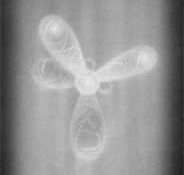Water molecule 'probability' electron shells - from theBigView.com

For those of us who are not well versed in the mysteries of QT, and perhaps would like to get a quick thumb sketch of what it's about, visit the 'big view' pages on topics from Relativity to the Uncertainty Principle: the Big View.

Here are some quotes from the Space and Time pages:

quote:

In the eyes of physics, the world used to be a predictable place. Aristotle and Ptolemy laid the foundation for the scientific understanding of the universe, which remained authoritative for one-and-a-half thousand years. Until the time of Galileo, the Greeks were undisputed in natural science and astronomy. Galileo, Copernicus, and Newton changed this. Isaac Newton (1642-1727) revolutionised physics with his proposition that all bodies are governed by the three laws of motion. The first law of motion states that a body continues in a state of rest or continues to be moving uniformly in a straight line unless a force is applied to the object. The second law states that the force applied to an object is proportional to its mass multiplied by acceleration (F=ma). The third law states that for every action there is an equal opposite reaction.

From Quantum Theory, it says:

quote:

Around 1900, Max Planck from the University of Kiel concerned himself with observations of the radiation of heated materials. He attempted to draw conclusions from the radiation to the radiating atom. On basis of empirical data, he developed a new formula which later showed remarkable agreement with accurate measurements of the spectrum of heat radiation. The result of this formula was so that energy is always emitted or absorbed in discrete units, which he called quanta. Planck developed his quantum theory further and derived a universal constant, which came to be known as Planck's constant. The resulting law states that the energy of each quantum is equal to the frequency of the radiation multiplied by the universal constant: E=f*h, where h is 6.63 * 10E-34 Js. The discovery of quanta revolutionised physics, because it contradicted conventional ideas about the nature of radiation and energy.

But the real issue is the Uncertainty Principle (as posted in the above by Israel), where the BV page says:

quote:

The uncertainty relation describes the "blur" between the measurable quantities of a particle in mathematical terms. Like much of the math in quantum theory, it is not for the faint of heart, which is to say it is completely unintelligible to most people.

And earlier on the QT page, it talked about measurement:

quote:

The nature of electrons seems odd. Seemingly they exist in different places at different points in time, but it is impossible to say where the electron will be at a given time. At time t1 it is at point A, then at time t2 it is at point B, yet without moving from A to B. It seems to appear in different places without describing a trajectory. Therefore, even if t1 and A can be pinpointed, it is impossible to derive t2 and B from this measurement. In other words: There seems to be no causal relation between any two positions.

But if no 'causality' can be ascribed to the observed, is it the fault of the electron's state in time, or that of the observational limitations in time? Both are questions "in time", and the crux of the dilemma in QT may be this paradox, that time as we understand it at the subatomic level is something else, subject to 'decay' and exists observationally only as a 'probability of time'. Or as Israel said above:

quote:

2.
When physicists studied the behavior of light, they came to the
conclusion that light ( light quanta) can sometime be a particle as a
“ mathematical point” and sometime a wave as a “ mathematical
wave". From behavior of light the SRT was born and here the particle
is also “ mathematical point”. It is hard to understand, why nobody think
about geometrical form of light quanta if it is real particle.
3.
For many years, physicists used Euclidian (static and firm ) geometry
for solving physical problems, and they thought there was only one geometry.
But Lobachevsky and Bolyai had another opinion.
They thought that to use only Euclidian geometry was not enough to
explain all the effects in the Universe. Why, because our Universe is not
static and firm. The physical processes in Universe change all the time
so the Euclidian geometry also has to change. This lead Lobachevsky and
Bolyai to discover Non-Euclidian geometry which is not static but elastic.

So it's the 'geometry' of time that is in question here. Is Relativistic 'time' the geometry to resolve this Cartesian problem? If the electron is both a wave and particle, geometrically both a point and an event in time, then perhaps how we do our measurements is the problem, rather than the solution... And that makes it 'paradoxical'.

But there is no easy solution to this, though the math behind QT makes it usable in our modern technology, and once mastered QT becomes 'easy'. If the quantum 'uncertainty' paradox is an artifact of how an electron diffracted is measured, though mathematically it is correct, then the solution must lie in a better experimental format than used to date. Perhaps gamma ray experiments, with tighter wavelength, would narrow down observational parameters?

Ivan

Also see: Uncertainty principle not so 'uncertain'...Ivan/solarcell fractals?
Posted From: 69.237.181.12
 Posted on Friday, October 31, 2008 - 12:29 am:

Can fractal geometry be used to improve solar cell efficiency?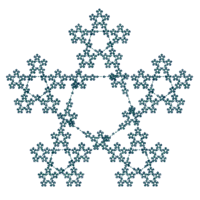(interactive - see: Von Koch curve example)
Fractal pentagram

Think how fractal geometry was used to improve cell phone antenna efficiency. So why can't this same idea be used to improve solar panel efficiency in generating electricity from sunlight?A solar cell silicon wafer (interactive - Wiki solar cells)

But how would it work on a relatively flat surface, unless these fractals were somehow integrated beneath the surface?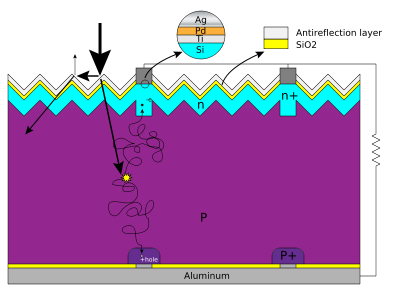Basic structure of a silicon based solar cell

Imagine a crystaline structure within the solar cell that looks like this: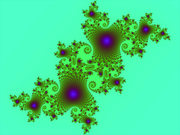Julia set, or perhaps a Mandelbrot set

Or like this:Animated construction of a Sierpinski Triangle, only going nine generations of infinite.

Can it be done to improve solar cell efficiency from the present, say 20-30% where the balance is lost into space, to something more like 50-60% efficiency? Can it be done inside the crystal, for example, where infinity is reduced into these fractal patterns?

For that matter, can any "infinite interrelationship" be reduced to its fractal geometry? Something to think about...An 'infinite' image made by fractal Sterling program: (sin(z) log(z) - tan(z))/(z2 cos(z) 2z) · c

Can this fractal geometry be applied to QT?

Ivan

 Topics | Last Day | Last Week | Tree View | Search | Help/Instructions | Program Credits Administration